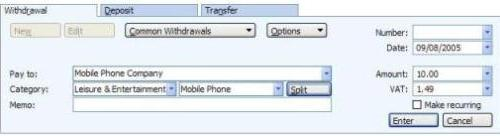# How VAT and GST are calculated

The way that VAT or GST in Microsoft Money is calculated might seem slightly confusing at first. You typically expect VAT to be added to the cost of a transaction, but it is not.

If you enter a transaction amount of £10, you might expect the VAT calculation (at 17.5%) to be £1.75 - however, this isn't correct.

The reason it's not correct is that the transaction total is £10, so the VAT in this case is £1.49. This is because the item (excluding VAT) cost is £8.51, making a sum of £10. If the net total was £10, then the gross total would be £11.75

The quick and easy calculation is
EX_VAT_TOTAL = WITH_VAT_TOTAL/(1+VAT_RATE)

Illustrating the point below is an automatic entry - the 'amount' box is the transaction total including VAT.Category: Tax

Keywords: GST, VAT